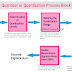A quantizer is a fundamental component in digital signal processing and communication systems. It is generally used to convert continuous analog signals into discrete digital values. The process of quantization involves taking a range of continuous input values and mapping them to a smaller set of discrete output values. Here, in this article, you can see the block diagram of a Quantizer or Quantization Process that will help you to understand the concept very easily.

## Quantizer or Quantization Process Block Diagram

Here, you can see the block diagram of a Quantizer or Quantization Process.

### Continuous Analog Signal Input

As we know the quantizer converts the analog signal into digital values. So the quantizer starts its work with a continuous analog signal as its input. This signal could represent various real-world phenomena like sound, images, or sensor readings.

### Determining the Quantization Levels

One of the most important and critical parameters in the quantization process is the determination of the number of discrete levels that the quantizer can represent. This is determined by the "bit depth" or "resolution" of the quantizer. For example, if you have a 3-bit quantizer, you will have 2^3 = 8 quantization levels. Each quantization level corresponds to a unique digital value.

### Defining the Quantization Range

In this stage, the entire range of the input analog signal is divided into smaller intervals according to the predetermined quantization levels. The size of these intervals depends on the quantization levels and the range of the input signal.

### Quantization Level Mapping

The input signal's amplitude within each interval is mapped to a specific quantization level. This mapping is done by comparing the input signal's amplitude with the boundaries of the intervals. The quantization level to which the signal belongs is the one that its amplitude is closest to.

### Discretization

After determining the quantization level, the quantizer assigns the corresponding digital value to the input sample. This digital value is typically represented in binary code that can be read easily in the form of digital numbers.

### Quantization Error

The Quantization process also introduces an error while generating digital values that is known as a quantization error. This error is the difference between the actual analog signal value and the quantized digital value. Smaller quantization intervals (more quantization levels) result in lower quantization errors, but they require more bits to represent each sample.

### Quantization Noise

It is a type of quantization error, which usually occurs when a continuous analog signal such as an analog audio signal is used to quantize into digital values. This noise happens because the quantizer has to round the analog signal's value to the nearest available digital value. This rounding introduces small differences between the original analog value and the quantized digital value.

### Signal-to-Noise Ratio (SNR)

This is associated with quality measurement. The quality of the quantization process is often measured using the Signal-to-Noise Ratio (SNR), which represents the ratio of the power of the original analog signal to the power of the quantization error. Higher SNR values indicate better quantization performance.

### Reconstruction

At the receiver's end, if necessary, the quantized digital values can be converted back into analog values through a process called reconstruction. This might involve using techniques like interpolation to approximate the original analog signal from the quantized values.

Understand Quantizer or Quantization Process with Block DiagramReviewed by Author on September 04, 2023 Rating: 5